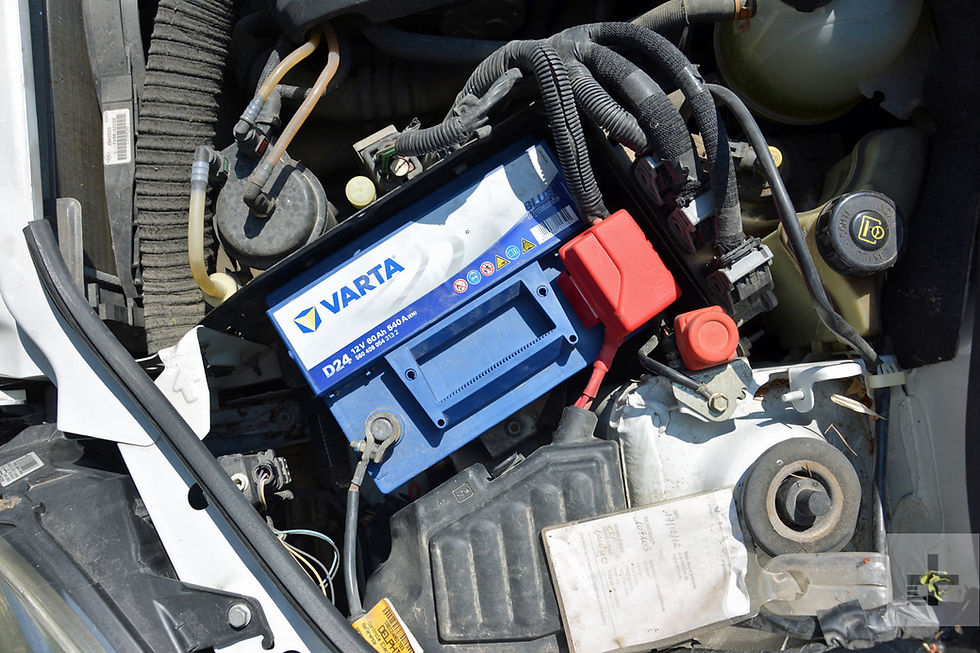top of page

Search

# What does Ah mean by on Car Battery | [33Ah 55Ah 65Ah 60Ah 85Ah]

Updated: May 23, 2022

Have you ever wondered what does ‘Ah’ rating of Car batteries mean? Here is something I want you to know about ‘Ah’ mentioned on car batteries. ‘Ah’ doesn’t mean the peak current battery can draw in one hour. But it is completely different from what most of the public think about the ‘Ah’ Rating.

In this blog, I will clear your lots of doubt about the rating of car batteries and all the information I embedded in this blog will make you an expert in front of your family and friend.

Related Posts:## What does Ah stand for on a Car battery?

The full form of ‘Ah’ mentioned on the battery is Ampere-hour and this refers to the capacity of a battery to provide continuous current over a specified period of time (that is 10 hours for class C10 battery and 20 Hours for class C20 battery) to a specific End of discharge Voltage (10.2V to 10.8V) at specific temperature before failing.

Whereas the End of discharge Voltage is the level to which the cell voltage is allowed to fall to before affecting the load.

As the battery starts discharging, the voltage across its terminals starts reducing too but the rate of voltage drop throughout the discharging period is not too high. The voltage battery provides before it fails is called End of discharge Voltage.

### Specification of [65Ah] car battery.

As per the industrial standard, Car batteries are generally 12V with a Class of C20. This means that the battery terminal voltage will be 12V and it will be guaranteed to provide continuous current equal to the ‘Ah’ rating of the battery divided by 20 over the discharge period of 20 hours.As shown in the above specification a 12V, 65Ah Class 20 battery is guaranteed to provide a continuous current of 3.25 Ampere over the discharge period of 20 hours (that is 3.25Ampere x 20Hour=65Ah) and the end of discharge voltage of the battery will be 10.2V at 27 Degree Celsius.

From the above specification of the 65 Ah car battery, it is also clear that if we completely discharge the battery over the time period of 10 hours, instead of 6.5 Ampere (65/10 = 6.5) of continuous current we will get only 5.59 Ampere of continuous current.

And if we compare the discharge current of the 65Ah battery for different discharge periods, we will find that the faster we discharge the battery the lesser backup we will get.

The same rule applies to all sizes and types of batteries.

Related Post: Car Battery Vs UPS Battery

## How much is the backup of the car battery?

The formula of electrical power stored in a battery is equal to the multiplication of its terminal voltage with its ampere-hour.

A 12V, 65Ah car battery can provide a continuous current of 3.25 Ampere for 20 hours. Hence it can provide backup for 20 hours for a constant load of 3.25 x 12 = 39 Watt.

Also from the specification of the 65Ah battery, we can see that for a discharge period of 10 hours, it will provide a maximum continuous current of 5.9A. Hence it can provide backup for 10 hours for a constant load of 5.9 x 12 = 70.8 Watt.

Now if we compare the backup of 65 Ah battery we will find that the maximum load for the backup of 10 hours [that is 70.8 Watt] is not equal to twice the maximum load for the backup of 20 hours [that is 39 Watt]

Similarly, we can calculate the maximum current as well as backup time for different Ah ratings [33Ah, 55Ah, 65Ah, 60Ah and 85Ah] of car batteries of class 20.

## 33Ah battery means

A 12V 33Ah Car battery is guaranteed to provide 1.65A [33/20=1.65A] of current for a discharge period of 20 Hours. And it can provide 20 hours of backup for a maximum load of 19.8 Watt [1.65x12=19.8].

## 55Ah battery means

A 12V 55Ah Car battery is guaranteed to provide 2.75A [55/20=2.75A] of current for a discharge period of 20 Hours. And it can provide 20 hours of backup for a maximum load of 33 Watt [2.75x12=33].

## 65Ah battery means

A 12V 65Ah Car battery is guaranteed to provide 3.25A [65/20=3.25A] of current for a discharge period of 20 Hours. And it can provide 20 hours of backup for a maximum load of 39 Watt [3.25x12=39].

## 60Ah battery means

A 12V 60Ah Car battery is guaranteed to provide 3A [60/20=3A] of current for a discharge period of 20 Hours. And it can provide 20 hours of backup for a maximum load of 36 Watt [3x12=36].

## 85Ah battery means

A 12V 85Ah Car battery is guaranteed to provide 4.25A [85/20=4.25A] of current for a discharge period of 20 Hours. And it can provide 20 hours of backup for a maximum load of 51 Watt [4.25x12=51].

3,974 views
bottom of page